American Journal of Operations Management and Information Systems
Volume 1, Issue 1, November 2016, Pages: 34-38

Strategic Decision Making in Portfolio Management with Goal Programming Model

Lam Weng Siew1, 2, *, Lam Weng Hoe1, 2

1Faculty of Science, Universiti Tunku Abdul Rahman, Kampar, Malaysia

2Centre for Mathematical Sciences, Centre for Business and Management, Universiti Tunku Abdul Rahman, Kampar, Malaysia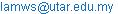(L. W. Siew)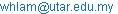(L. W. Hoe)

*Corresponding author

Lam Weng Siew, Lam Weng Hoe. Strategic Decision Making in Portfolio Management with Goal Programming Model. American Journal of Operations Management and Information Systems. Vol. 1, No. 1, 2016, pp. 34-38. doi: 10.11648/j.ajomis.20160101.14

Received: October 23, 2016; Accepted: November 12, 2016; Published: December 12, 2016

Abstract: Enhanced index tracking is a popular type of portfolio management which aims to construct the optimal portfolio in order to generate higher portfolio mean return than the benchmark index mean return. Enhanced index tracking is a dual objective optimization problem which can be represented by a goal programming model to determine the trade-off between maximizing the portfolio mean return and minimizing the tracking error. The objective of this paper is to apply the goal programming model in constructing the optimal portfolio to track the Technology Index in Malaysia. In this study, the data consists of weekly return of the companies from technology sector in Malaysia stock market. The results of this study indicate that the optimal portfolio is able to outperform Technology Index by generating weekly excess mean return 0.3798% at minimum tracking error 2.0980%. The significance of this study is to identify and apply the goal programming model as a strategic decision-making tool for the fund managers to track the benchmark Technology Index effectively in Malaysia stock market.

Keywords: Goal Programming Model, Enhanced Index Tracking, Optimal Portfolio, Mean Return, Tracking Error

Contents

1. Introduction

At the operational level, many decisions are made in order to achieve the desired outcome that contribute to the achievement of a company’s overall strategic goal. In operations management, there are quantitative models, optimization models and other techniques available which help the managers to make better decisions scientifically. In portfolio management, the fund managers have to determine the optimal portfolio which can generate higher return at minimum risk of loss. Enhanced index tracking is a popular type of portfolio management which aims to construct the optimal portfolio in order to generate higher portfolio mean return than the benchmark index mean return at minimum tracking error . Tracking error is a risk measure of how closely the portfolio return follows the benchmark index return .

Tracking the sectorial index is important because the index represents the overall performance of the economic sectors in a country such as technology sector, construction sector and industrial product sector. In tracking the benchmark index, the optimization model with goal programming approach has been developed to determine the trade-off between minimizing the tracking error and maximizing the mean return of the portfolio . The optimization model has been studied by different researchers as a strategic decision-making tool in portfolio management [4-9]. Tracking error and mean return of the optimal portfolio are two elements in enhanced index tracking problem [10,11]. The objective of this paper is to apply the optimization model with goal programming approach in constructing the optimal portfolio to track the Technology Index in Malaysia. The performance of the optimal portfolio is then compared with the benchmark Technology Index. The rest of the paper is organized as follows. The next section describes the materials and methods used in this study. Section 3 presents the empirical results of this study. Section 4 concludes the paper.

2. Materials and Methods

2.1. Data

In this study, the data consists of weekly return of the stocks from technology sector which are listed on the Malaysia stock market as shown in Table 1 .

Table 1. List of Stocks from Technology Sector on Malaysia Stock Market.

 Stocks CUSCAPI D&O DATAPRP DIGISTAR DNEX GHLSYS GPACKET GTRONIC MPI MSNIAGA NOTION OMESTI PANPAGE PENTA THETA TRIVE UNISEM

The study period is from January 2012 until December 2015. This data is applied in the optimization model with goal programming approach for portfolio construction to track the Technology Index in Malaysia. In portfolio construction with the goal programming model, the decision variables represents the optimal portfolio composition that can be determined by solving the model . The return of the stocks is determined as below .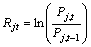(1)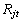is the return of stock j at time t,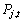is the closing price of stock j at time t,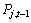is the closing price of stock j at time t-1.

The return of the benchmark index is determined as below .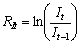(2)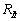is the return of index at time t,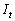is the index value at time t,is the index value at time t-1.

The mean return of the stock j is calculated as below .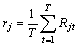(3)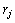is the mean return of stock j,is the return of stock j at time t,

T is the number of observations.

Figure 1 shows the construction process of the optimal portfolio in tracking the benchmark Technology Index with an optimization model .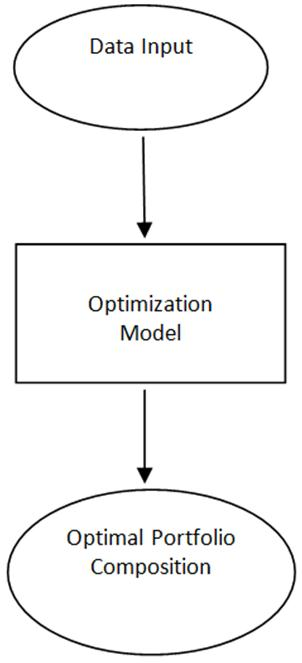Figure 1. Construction Process of the Optimal Portfolio Composition with Optimization Model.

In this study, the optimization model with goal programming approach is solved with LINGO software .

2.2. Goal Programming Model

In enhanced index tracking, there are two goals to be achieved, which are minimizing the tracking error and maximizing the mean return of the optimal portfolio. Reference  proposed the dual objective optimization model for enhanced index tracking problem which is formulated as follow.

Minimize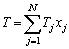(4)

Maximize(5)

subject to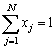(6)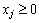(7)is the mean return of stock j in the optimal portfolio,

N is the number of stocks,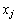is the weight of stock j in the optimal portfolio,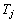is the tracking error of stock j,

T is the portfolio tracking error,

R is the portfolio mean return,

Equation (4) is the first goal which minimizes the portfolio tracking error. Equation (5) is the second goal which maximizes the portfolio mean return. Constraint (6) ensures that the total weights of stocks invested equal to one. Constraint (7) ensures that the weight of each stock j in the optimal portfolio are positive.

The dual objective optimization model above is solved with goal programming approach . Goal programming is able to handle decision problems that involve multiple goals . The goal programming model is formulated as follow.

Minimize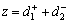(8)

subject to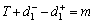(9)(10)(11)(12)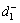is the extent of underachievement for tracking error,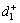is the extent of overachievement for tracking error,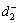is the extent of underachievement for portfolio mean

return,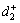is the extent of overachievement for portfolio mean

return,

T is the portfolio tracking error,

R is the portfolio mean return,

m is target value for portfolio tracking error,

n is target value for portfolio mean return,

N is the number of stocks,is the weight of stock j in the optimal portfolio,

Equation (8) is the objective function of the model which minimizes the sum of deviations of all decision goals. Equation (9) is the first goal which minimizes the portfolio tracking error. Equation (10) is the second goal which maximizes the portfolio mean return. Constraint (11) ensures that the total weights of stocks invested equal to one. Constraint (12) ensures that the weight of each stock j in the optimal portfolio are positive.

2.3. Portfolio Performance

Tracking error and mean return of the optimal portfolio are two elements in enhanced index tracking problem [10,11]. Tracking error is the standard deviation of the difference between the returns of the portfolio and the returns of the benchmark index [17-19]. The formula for tracking error is as follows.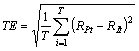(13)

TE is the tracking error,

T is the number of periods,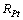is the mean return of the optimal portfolio at time t,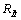is the mean return of the benchmark index at time t.

The mean return of the optimal portfolio is formulated as follow [14,20].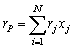(14)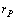is the mean return of the optimal portfolio,is the weight of stock j in the optimal portfolio,is the mean return of stock j in the optimal portfolio. Excess return is defined as the difference between the portfolio mean return and benchmark index mean return which is formulated as follow [3, 10].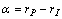(15)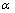is the excess return,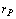is the mean return of the optimal portfolio,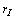is the mean return of the benchmark index.

The performance of the optimal portfolio is measured with information ratio [10,21]. The information ratio is defined as the ratio of portfolio’s excess mean return to the portfolio’s tracking error which is formulated as below.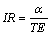(16)

IR is the information ratio,is the excess mean return of the optimal portfolio over the mean return of the benchmark index return,

TE is the tracking error.

Higher information ratio indicates higher performance of the optimal portfolio.

3. Empirical Results

Table 2 displays the summary statistics of the stocks returns in this study.

Table 2. Summary Statistics of the Stocks Returns.

 Stocks Mean Standard Deviation CUSCAPI -0.0052 0.0723 D&O 0.0035 0.0660 DATAPRP -0.0034 0.0526 DIGISTAR -0.0035 0.0540 DNEX -0.0009 0.0549 GHLSYS 0.0059 0.1426 GPACKET -0.0042 0.0682 GTRONIC 0.0106 0.0393 MPI 0.0063 0.0460 MSNIAGA -0.0036 0.0343 NOTION -0.0081 0.0625 OMESTI -0.0018 0.0513 PANPAGE -0.0013 0.0357 PENTA 0.0051 0.0590 THETA -0.0031 0.0958 TRIVE -0.0061 0.1091 UNISEM 0.0035 0.0543

As reported in Table 2, the stocks returns show that the values of mean and standard deviation are different for each stock in this period of study. GTRONIC gives the highest mean return at 0.0106 whereas GHLSYS gives the highest standard deviation at 0.1426.

Table 3 presents the stock selection in optimal portfolio which is constructed by solving the goal programming model.

Table 3. Stock Selection in Optimal Portfolio.

 Stocks Weights (%) CUSCAPI 0.32 D&O 1.81 DATAPRP 0.48 DIGISTAR 0.00 DNEX 4.21 GHLSYS 2.68 GPACKET 0.00 GTRONIC 29.24 MPI 21.20 MSNIAGA 0.00 NOTION 0.00 OMESTI 0.00 PANPAGE 0.00 PENTA 14.53 THETA 0.00 TRIVE 1.65 UNISEM 23.88

As shown in Table 3, the list of stocks with positive weights indicate that those stocks are selected by the goal programming model in constructing the optimal portfolio to track the Technology Index in Malaysia. The optimal portfolio consists of 10 stocks with different weights in tracking the Technology Index. DIGISTAR, GPACKET, MSNIAGA, NOTION, OMESTI, PANPAGE and THETA are not selected in the optimal portfolio because these stocks give the weights of 0.00%.

Figure 2 presents the optimal portfolio composition with goal programming model.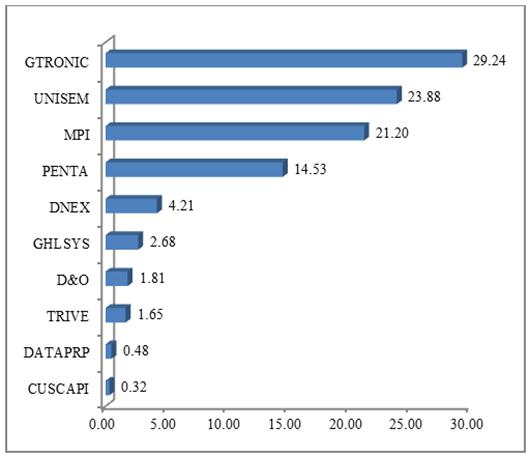Figure 2. Optimal Portfolio Composition with Goal Programming Model.

As shown in Figure 2, the optimal portfolio consists of CUSCAPI (0.32%), D&O (1.81%), DATAPRP (0.48%), DNEX (4.21%), GHLSYS (2.68%), GTRONIC (29.24%), MPI (21.20%), PENTA (14.53%), TRIVE (1.65%) and UNISEM (23.88%). This implies that the optimal portfolio composition are the optimal solution of the goal programming model. GTRONIC is the most dominant stock in the optimal portfolio with 29.24% of the allocated fund. On the other hand, CUSCAPI is the smallest stock in the optimal portfolio with 0.32% of the allocated fund.

Table 4 and Figure 3 present the summary statistics and return distribution of the optimal portfolio of the goal programming model respectively.

Table 4. Summary Statistics of the Optimal Portfolio.

 Goal Programming Model Summary Statistics Portfolio Mean Return 0.006068 Portfolio Standard Deviation 0.033702 Portfolio Skewness -0.259044 Portfolio Kurtosis 1.356789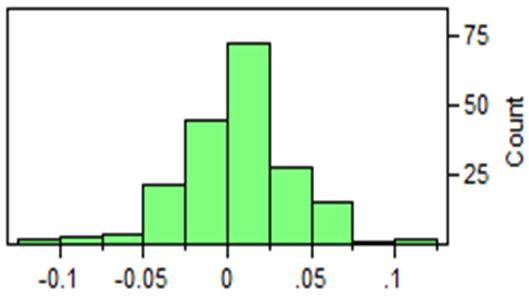Figure 3. Portfolio Return Distribution of the Goal Programming Model.

Based on Table 4 and Figure 3, the optimal portfolio of goal programming model gives the mean return 0.006068 (0.6068%) with standard deviation 0.033702. Besides that, the skewness and kurtosis value of the optimal portfolio are

-0.259044 and 1.356789 respectively.

Table 5 displays the performance of the optimal portfolio constructed using the goal programming model.

Table 5. Performance of the Optimal Portfolio of Goal Programming Model.

 Portfolio Technology Index Goal Programming Model Mean Return (%) 0.2270 0.6068 Excess Return (%) - 0.3798 Tracking Error (%) - 2.0980 Information Ratio - 0.1810

As shown in Table 5, the weekly mean return for Technology Index is 0.2270% based on the study period. The optimal portfolio tracks the Technology Index with weekly mean return 0.6068% which is higher than the mean return of Technology Index. This implies that the optimal portfolio constructed by the goal programming model is able to outperform the Technology Index with weekly excess mean return 0.3798% at minimum tracking error 2.0980%. Besides that, the information ratio 0.1810 indicates that the optimal portfolio can generate weekly excess mean return 0.1810% over the mean return of Technology Index at 1% tracking error. Therefore, the goal programming model is suitable to be used as a strategic decision-making tool for the fund managers and investors in Malaysia.

4. Conclusion

This paper discusses about the strategic decision-making tool in portfolio management by using the goal programming model to track the Technology Index in Malaysia. In conclusion, the optimal portfolio constructed by the goal programming model is able to outperform Technology Index by generating weekly excess mean return 0.3798% at minimum tracking error 2.0980%. The significance of this study is to identify and apply the goal programing model as a strategic decision-making tool for the fund managers and investors to track the benchmark Technology Index effectively in Malaysia.

References

1. J. E. Beasley, N. Meade, and T. J. Chang, "An evolutionary heuristics for the index tracking problem," European Journal of Operational Research, vol. 148, pp. 621-643, 2003.
2. R. Roll, "A mean variance analysis of tracking error," The Journal of Portfolio Management, vol. 18, pp. 13-22, 1992.
3. L.C. Wu, S. C. Chou, C. C. Yang, and C. S. Ong, "Enhanced Index Investing Based on Goal Programming," The Journal of Portfolio Management, vol. 33, pp. 49-56, 2007.
4. N. A. Canakgoz, and J. E. Beasley, "Mixed integer programming approaches for index tracking and enhanced indexation," European Journal of Operational Research, vol. 196, pp. 384-399, 2008.
5. G. Guastaroba, and M. G. Speranza, "Kernel Search: An application to index tracking problem," European Journal of Operational Research, vol. 217, pp. 54-68, 2012.
6. W. S. Lam, J. Saiful, and I. Hamizun, "The impact of different economic scenarios towards portfolio selection in enhanced index tracking problem," Advanced Science Letters, vol. 21, no. 5, pp. 1285-1288, 2015.
7. W. S. Lam, J. Saiful, and I. Hamizun, "An empirical comparison of different optimization models in enhanced index tracking problem," Advanced Science Letters, vol. 21, no. 5, pp. 1278-1281, 2015.
8. W. S. Lam, J. Saiful, and I. Hamizun, "The impact of human behavior towards portfolio selection in Malaysia." Procedia of Social and Behavioral Sciences, vol. 172, pp. 674-678, 2015.
9. N. Meade, and J. E. Beasle, "Detection of momentum effects using an index out-performance strategy," Quantitative Finance, vol. 11, no. 2, 313-326, February 2011.
10. L. C. Wu, and L. H. Wu, "Tracking a benchmark index – using a spreadsheet-based decision support system as the driver," Expert Systems, vol. 30, pp. 79-88, 2012.
11. W. S. Lam, and W. H. Lam, "Portfolio optimization for index tracking problem with mixed integer programming model," Journal of Scientific Research and Development, vol. 2, no. 10, pp. 5-8, 2015.
12. Bursa Malaysia, n.d.. Company Announcements | Bursa Malaysia Market. [online] Available at: <
http://www.bursamalaysia.com/market/securities/equities/prices/#/?filter=BS08&board=MAIN-MKT&sector=TECHNOLOGY&page=1>
13. L. J. Gitman, M. D. Joehnk, and L. J. Smart, Fundamentals of Investing, 11th ed, Pearson, 2011.
14. W. S. Lam, and W. H. Lam, "Selection of mobile telecommunications companies in portfolio optimization with mean-variance model," American Journal of Mobile Systems, Applications and Services, vol. 1, no. 2, pp. 119-123, 2015.
15. LINGO, Version 12. Chicago: LINDO Systems Inc, 2010.
16. H. A. Taha, Operations Research:An Introduction. 9th ed, New Jersey, Prentice Hall, 2011.
17. W. S. Lam, J. Saiful, and I. Hamizun, "Comparison between Two Stage Regression Model and Variance Model in Portfolio Optimization," Journal of Applied Science and Agriculture, vol. 9, no. 18, pp. 36-40, 2014.
18. W. S. Lam, J. Saiful, and I. Hamizun, "Index tracking modelling in portfolio optimization with mixed integer linear programming," Journal of Applied Science and Agriculture, vol. 9, no. 18, pp. 47-50, 2014.
19. N. Meade, and G. R. Salkin, "Developing and Maintaining an Equity Index Fund," Journal of Operation Research Society, vol. 41, no. 7, pp. 599-607, 1990.
20. F. K. Reilly, and K. C. Brown, Investment Analysis and Portfolio Management. 10th Ed, Mason, South Western Cengage Learning, 2012.
21. C. L. Israelsen, "A Refinement to the Sharpe Ratio and Information Ratio," Journal of Asset Management, vol. 5, no. 6, pp. 423-427, 2005.

 Contents 1. 2. 2.1. 2.2. 2.3. 3. 4.
Article ToolsAbstractPDF(230K)